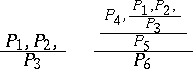# Derivation tree

(diff) ← Older revision | Latest revision (diff) | Newer revision → (diff)
A notation for derivations (cf. Derivation, logical) in a calculus, in which the elements of the derivations from whichis obtained by one application of a derivation rule are written down above each element. For instance, the derivation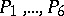in which,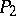and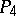are axioms,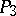is obtained by one application of some rule from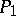and, andis obtained fromand, while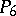is obtained fromand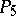, may be written down as follows: